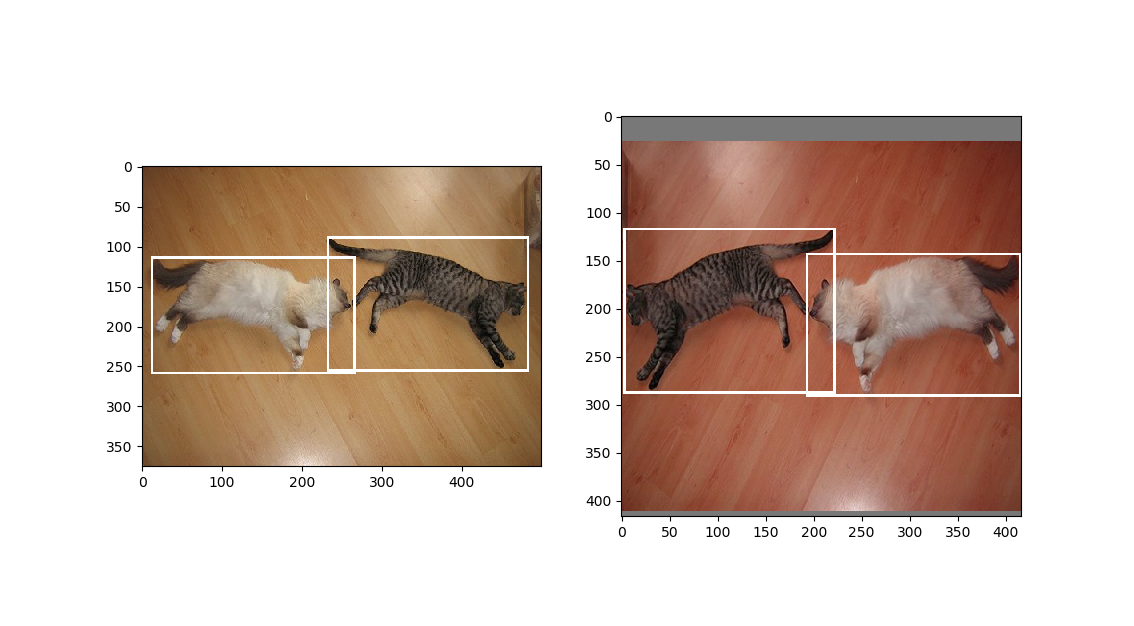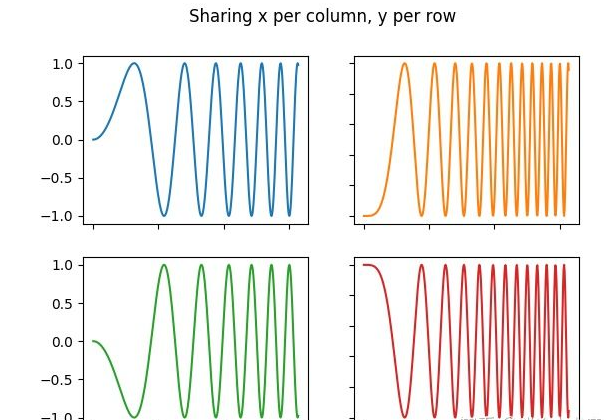• Matplotlib在一张画布上多个种方法，plt.subplot,plt.subplots。 目录回顾plt.subplots()画法plt.subplot()画法保存 回顾 之前也用过plt.subplots()在一张过多个，今天看到用plt.subplot()的画法想...
• arg1: 在垂直方向同时张图 arg2: 在水平方向同时张图 arg3: 当前命令修改的是第几张图 plt.figure()另起一张新的画布 from PIL import Image import matplotlib.pyplot as plt image1 = Image.open('1....
subplot(arg1, arg2, arg3)
arg1: 在垂直方向同时画几张图 arg2: 在水平方向同时画几张图 arg3: 当前命令修改的是第几张图
	plt.figure()另起一张新的画布

from PIL import Image
import matplotlib.pyplot as plt

image1 = Image.open('1.jpg')
image2 = Image.open('2.jpg')

plt.subplot(121)
plt.imshow(image1)
plt.subplot(122)
plt.imshow(image2)
plt.show()展开全文• 展开全部python matplotlib模块 如何画两张图出2113来的方法：5261代码如下所示：import numpy as npimport matplotlib.pyplot as plt#创建自变量4102数组x= np.linspace(0,2*np.pi,500)#创建函数值数组y1 = np.sin...
展开全部
python matplotlib模块 如何画两张图出2113来的方法：5261
代码如下所示：
import numpy as np
import matplotlib.pyplot as plt
#创建自变量4102数组
x= np.linspace(0,2*np.pi,500)
#创建函数值数组
y1 = np.sin(x)
y2 = np.cos(x)
y3 = np.sin(x*x)
#创建图形
plt.figure(1)
'''
意思是在1653一个2行2列共4个子图的图中，定位第1个图来进行操作（画图）。
最后面那个1表示第1个子图。那个数字的变化来定位不同的子图
'''
#第一行第一列图形
ax1 = plt.subplot(2,2,1)
#第一行第二列图形
ax2 = plt.subplot(2,2,2)
#第二行
ax3 = plt.subplot(2,1,2)
#选择ax1
plt.sca(ax1)
#绘制红色曲线
plt.plot(x,y1,color='red')
#限制y坐标轴范围
plt.ylim(-1.2,1.2)
#选择ax2
plt.sca(ax2)
#绘制蓝色曲线
plt.plot(x,y2,'b--')
plt.ylim(-1.2,1.2)
#选择ax3
plt.sca(ax3)
plt.plot(x,y3,'g--')
plt.ylim(-1.2,1.2)
plt.show()附上效果图。
展开全文• 接触了python之后，发现可以用来地理中的一些图片，就真是振奋人心的好消息。在我们今天子图的内容中，也会提到卫星弧段。感兴趣的小伙伴我们一起来看看吧。Python可通过subplot函数多个子。调用形式如：s...
开始讲今天的内容之前，先说一点题外话。上学的时候地理课上会画一些等高图、小地图之类的，那时候小编就对地理产生了浓厚的兴趣，不过最后因为种种原因还是没有选择继续学习地理方面的知识了。接触了python之后，发现可以用来画地理中的一些图片，就真是振奋人心的好消息。在我们今天画子图的内容中，也会提到卫星弧段图。感兴趣的小伙伴我们一起来看看吧。
Python可通过subplot函数画多个子图。调用形式如：subplot(nrows,ncols,index)，图表的整个绘图区域被分成nrows行和ncols列，按照从左到右，从上到下的顺序对每个子区域进行编号，左上的子区域编号为1。index参数指定创建的Axes对象所在的区域。
matplotlib官网上的一个2*2多子图例子如下所示：import matplotlib.pyplot as plt
import numpy as np
# Some example data to display
x = np.linspace(0, 2 * np.pi, 400)
y = np.sin(x ** 2)
fig, ((ax1, ax2), (ax3, ax4)) = plt.subplots(2, 2)
fig.suptitle('Sharing x per column, y per row')
ax1.plot(x, y)
ax2.plot(x, y**2, 'tab:orange')
ax3.plot(x, -y, 'tab:green')
ax4.plot(x, -y**2, 'tab:red')
for ax in fig.get_axes():
ax.label_outer()
比如要将卫星弧段图和SNR图两个图按照上下顺序放置于同一张图时，可采用如下方式：import matplotlib.pyplot as plt
plt.figure()
plt.subplot(211)
plt.plot(t1, Satarc)
plt.subplot(212)
plt.plot(t2, SNR)
以上就是python中用subplot画多个子图的方法，同时我们也可以把把它们画在一个图中。更多有趣用python画地理图片的小方法，小伙伴们也可以自行探索。希望今天的内容能对大家有所帮助~
展开全文• 我在matplotlib上找到了以下示例:import numpy as npimport matplotlib.pyplot as pltx1 = np.linspace(0.0, 5.0)x2 = np.linspace(0.0, 2.0)y1 = np.cos(2 * np.pi * x1) * np.exp(-x1)y2 = np.cos(2 * np.pi * x2)...
我在matplotlib上找到了以下示例:
import numpy as np
import matplotlib.pyplot as plt
x1 = np.linspace(0.0, 5.0)
x2 = np.linspace(0.0, 2.0)
y1 = np.cos(2 * np.pi * x1) * np.exp(-x1)
y2 = np.cos(2 * np.pi * x2)
plt.subplot(2, 1, 1)
plt.plot(x1, y1, 'ko-')
plt.title('A tale of 2 subplots')
plt.ylabel('Damped oscillation')
plt.subplot(2, 1, 2)
plt.plot(x2, y2, 'r.-')
plt.xlabel('time (s)')
plt.ylabel('Undamped')
plt.show()
我的问题是:为了使地块并排，我需要更改什么？
参考方案
将子图设置更改为:
plt.subplot(1, 2, 1)
...
plt.subplot(1, 2, 2)
subplot的参数是:行数，列数以及当前正在使用的子图。因此1, 2, 1的意思是“一个1行2列图形:转到第一个子图。”然后1, 2, 2表示“1行2列图形:转到第二个子图”。
当前，您正在要求2行1列(即一个在另一个之上)的布局。您需要改用1行2列的布局。当您这样做时，结果将是:为了最大程度地减少子图的重叠，您可能需要加入:
plt.tight_layout()
演出前。屈服:Matplotlib'粗体'字体 - python
跟随this example：import numpy as np import matplotlib.pyplot as plt fig = plt.figure() for i, label in enumerate(('A', 'B', 'C', 'D')): ax = f…Matplotlib-固定x轴缩放比例和自动缩放y轴 - python
我只想绘制部分数组，固定x部分，但让y部分自动缩放。我尝试如下所示，但是它不起作用。有什么建议么？import numpy as np import matplotlib.pyplot as plt data=[np.arange(0,101,1),300-0.1*np.arange(0,101,1)] plt.figure() plt.scatter(da…在返回'Response'(Python)中传递多个参数 - python
我在Angular工作，正在使用Http请求和响应。是否可以在“响应”中发送多个参数。角度文件：this.http.get("api/agent/applicationaware").subscribe((data:any)... python文件：def get(request): ... return Response(seriali…Python exchangelib在子文件夹中读取邮件 - python
我想从Outlook邮箱的子文件夹中读取邮件。Inbox ├──myfolder 我可以使用account.inbox.all()阅读收件箱，但我想阅读myfolder中的邮件我尝试了此页面folder部分中的内容，但无法正确完成https://pypi.python.org/pypi/exchangelib/ 参考方案 您需要首先掌握Folder的myfo…Python GPU资源利用 - python
我有一个Python脚本在某些深度学习模型上运行推理。有什么办法可以找出GPU资源的利用率水平？例如，使用着色器，float16乘法器等。我似乎在网上找不到太多有关这些GPU资源的文档。谢谢！ 参考方案 您可以尝试在像Renderdoc这样的GPU分析器中运行pyxthon应用程序。它将分析您的跑步情况。您将能够获得有关已使用资源，已用缓冲区，不同渲染状态上…
展开全文• 目录1，问题背景2，在一张中显示多条曲线3，在一个窗口显示多张图片注意：1，问题背景在一次任务中，老师给出了几个公式，其中含有三个自变量λ、μ、c。因为要观察最终的结果受这三个变量的影响，所以采用如下的...
• lns1 = ax1.plot(np.arange(n_epoch), fig_loss, label="Loss") ax1.set_xlabel('iteration') ax1.set_ylabel('training loss') # 训练和验证种准确率曲线放在一张中 fig2, ax2 = plt.subplots() ax3 = ax2....
• import matplotlib.pyplot as plt import matplotlib as mpl import numpy as np mpl.rcParams["font.sans-serif"] = ["SimHei"] mpl.rcParams["axes.unicode_minus"] = False x = np.arange(4) bar_width = 0.1...mysql nosql
• ## plt绘制多个图片

千次阅读 2020-08-17 16:25:40
plt.figure() plt.subplot(2,1,1) plt.plot(train_data['outtemp'][0:101]) plt.subplot(2,1,2) plt.plot(train_data['temperature'][0:101]) plt.show()可视化
• import matplotlib.pyplot as plt import matplotlib as mpl import numpy as np import pandas as pd # 解决中文显示问题 mpl.rcParams['font.sans-serif'] = ['SimHei'] mpl.rcParams['axes.unicode_minus'] = ...
• plt.subplt(行，列，第几个图)函数定义要那张子图，其中行和列定义要张图，如plt.subplot(2,3,1)就是定义大图含有2行3列子图，就是6张图，第三位的1表示开始第一张图，因此后面的图一次就是plt.subplot(2,3,...
• 在一张画布上多个子, 尝试种方式: plt.subplots(nrows, ncols) 子图需要一个一个的指定 plt.subplot(nrows, ncols, i) 子图可以使用for循环 一. plt.subplots() fig, axes = plt.subplots(nrows = 3, ncols ...python
• 循环使用plt.savefig()保存图片时，出现前面的图像和此步要的图像都在一个画布中的情况 解决办法： 在绘图前加入 plt.figure() 创建一个新的figure对象， 这样就不会因为循环绘图而把在一个画布中 .....python
• 我在同时画两个图形时遇到了一些麻烦，没有在一个中显示出来。但是根据文档，我编写了代码，只有1显示。我想也许我失去了一些重要的东西。有谁能帮我弄清楚吗？谢谢。（代码中使用的*tlist_first*是一个数据列表...
• Opencv查找两张图片不同的部分以及图片中特定的像素替换 Opencv识别两张图片的不同部分demo： import cv2 import numpy as np from matplotlib import pyplot as plt import argparse def matchAB(fileA, fileB): ...
• I found the following example on matplotlib:import numpy as npimport matplotlib.pyplot as pltx1 = np.linspace(0.0, 5.0)x2 = np.linspace(0.0, 2.0)y1 = np.cos(2 * np.pi * x1) * np.exp(-x1)y2 = np.cos(2 ...
• 文章目录一、 fig, ax = plt.subplots()的作用二、参数的含义三、上排列多个子图四、把多个子图一起合并到一个上五、画图刻度、图例等字体、字体大小、刻度密度、线条样式设置 一、 fig, ax = plt.subplots()的...python 数据可视化
• 因为想要对比两张图的，所以需要同时绘出 但matplotlib在遇到plt.show()函数在阻塞模式时会挂起， 代码并不会继续运行，这就导致只能一张一张的显示，不方便对比。 解决方法 利用plot.ion()函数，调节到交互模式具体...
• 一个figure对象包含了多个子，可以使用subplot（）函数来绘制子图： subplot（numbRow ， numbCol ，plotNum ） 或者 subplot(numbRow numbCol plotNum) ... plt.figure(figsize=(15,15)) ..
• 要实现两张图片的简单拼接,必须计算homography矩阵（单应性矩阵），矩阵的计算至少需要在中找到四个相似的点，用这个矩阵把那张图片变换后放到另一张图片相应的位置 ( 就是相当于把两张图片中定好的四个相似的点給...opencv
• 话对比，如果个数量级的纵坐标，我们要进行趋势比较，放在同一坐标轴，某一个往往被压缩的的很小。所以需要左右双Y轴画图。 1.随便获取数列，对应的索引化成一样就可以了，下面是量化的数据处理，可以不用看，...
• 刚开始，找几个教程，呕吼，简单，plt.*** 一顿写。完了。遇到坐标啊之类的细节不会调？ 打开谷哥，要啥搜啥。 我最开始觉得这样蛮好的，这是一个很容易学的lib嘛。 然后问题出现了，matplotlib最烦人的地方...
• 许多场合下，我们需要将条甚至多条曲线放置在一张中进行对比，matplotlib可以轻松实现该要求。 完整代码如下： import matplotlib.pyplot as plt import numpy as np data = np.arange(0,1,0.01) #伪造从0到1以...python 可视化 matplotlib
• 1.plt.pie绘制扇形 # 1. plt.pie绘制扇形 import matplotlib.pyplot as plt %matplotlib inline labels = 'frogs','hogs','dogs','logs' sizes = 15,20,45,10 colors = 'yellowgreen','gold','...
• plt.plotfig = plt.figure(figsize=(16,16),dpi=300) # 初始化一张画布，可以设置figsize和dpiplt.plot() #直接在一张...设计模式 多线程 redis nginx js...